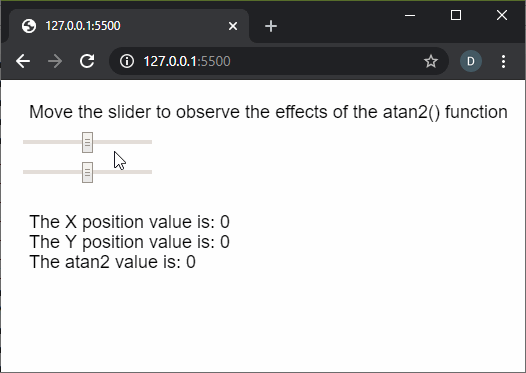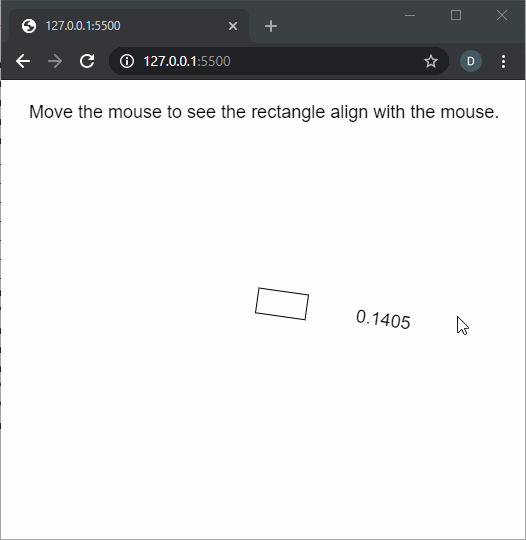GFG App
Open AppBrowser
Continue

The atan2() function in p5.js is used to calculate the angle from a specified point of origin measured from the positive x-axis. The values are returned in the range of PI to -PI as floating points. It is commonly used for orienting geometry to the position of the cursor.

Syntax:

`atan2(y, x)`

Parameters: This function accepts two parameters as mentioned above and described below.

• y: It is a number that specifies the y-coordinate of the point.
• x: It is a number that specifies the x-coordinate of the point.

Return Value: It returns a number that denotes the arc tangent of the given point.

Below examples illustrate the atan2() function in p5.js:

Example 1:

 `function` `setup() { ` `  ``createCanvas(500, 200); ` `  ``textSize(18); ` ` `  `  ``text(``"Move the slider to observe the effects"` `         ``+ ``" of the atan2() function"``, 20, 30); ` ` `  `  ``sliderXPos = createSlider(-200, 200, 0, 1); ` `  ``sliderXPos.position(20, 50); ` ` `  `  ``sliderYPos = createSlider(-200, 200, 0, 1); ` `  ``sliderYPos.position(20, 80); ` `} ` ` `  `function` `draw() { ` `  ``clear(); ` `  ``text(``"Move the slider to observe the effects"` `         ``+ ``" of the atan2() function"``, 20, 30); ` ` `  `  ``sliderXVal = sliderXPos.value(); ` `  ``sliderYVal = sliderYPos.value(); ` ` `  `  ``atan2Val = atan2(sliderXVal, sliderYVal); ` ` `  `  ``text(``"The X position value is: "` `+ sliderXVal, 20, 140); ` `  ``text(``"The Y position value is: "` `+ sliderYVal, 20, 160); ` `  ``text(``"The atan2 value is: "` `+ atan2Val, 20, 180); ` `} `

Output:Example 2:

 `function` `setup() { ` `  ``createCanvas(500, 400); ` `  ``textSize(18); ` `  `  `  ``text(``"Move the mouse to see the rectangle"` `        ``+ ``" align to it."``, 20, 30); ` `} ` `  `  `function` `draw() { ` `  ``clear(); ` `  ``text(``"Move the mouse to see the rectangle"` `        ``+ ``" align with the mouse."``, 20, 30); ` `  `  `  ``// Move the rectangle to the ` `  ``// middle of the screen ` `  ``translate(width / 2, height / 2); ` `  `  `  ``// Use the atan2() function to find ` `  ``// the value according to the mouse ` `  ``// coordinates ` `  ``let adjustedValue = atan2(mouseY - height / 2, ` `                            ``mouseX - width / 2); ` `  ``rotate(adjustedValue); ` `  `  `  ``// Draw a rectangle ` `  ``rect(0, 0, 50, 25); ` `  `  `  ``text(adjustedValue.toFixed(4), 100, 20); ` `} `

Output:Online editor: https://editor.p5js.org/

Reference: https://p5js.org/reference/#/p5/atan2

My Personal Notes arrow_drop_up